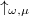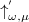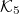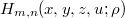﻿

### Lie algebra representations and 2-index 4-variable 1-parameter Hermite polynomials

#### Abstract

This paper is an attempt to stress the usefulness of multi-variable special functions by expressing them in terms of the corresponding Lie algebra or Lie group. The problem of framing the 2-index 4-variable 1-parameter Hermite polynomials (2I4V1PHP) into the context of the irreducible representations$\uparrow _{\omega,\mu}$ of$\mathcal{G}(0,1)$ and$\uparrow^{'} _{\omega,\mu}$ of$\mathcal{K}_{5}$ is considered. Certain relations involving 2I4V1PHP$H_{m,n}(x,y,z,u;\rho)$ are obtained using the approach adopted by Miller. Certain examples involving other forms of Hermite polynomials are derived as special cases. Further, some properties of the 2I4V1PHP$H_{m,n}(x,y,z,u;\rho)$ are obtained by using a quadratic combination of four operators defined on a Lie algebra of endomorphisms of a vector space.

DOI Code: 10.1285/i15900932v39n1p65

Keywords: 2-index 4-variable 1-parameter Hermite polynomials; Lie group; Lie algebra; representation theory; implicit formulae

Full Text: PDF